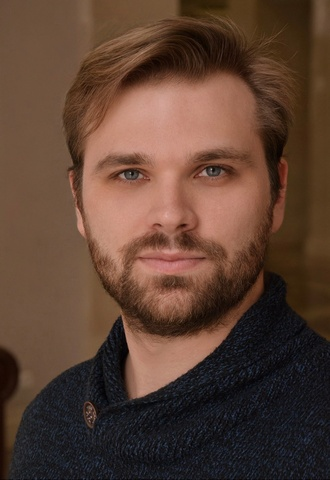# Andrey Selutin

### ScheduleWinner of international competition

Andrey SelutinВ is a graduate in voice of the MichaЕ‚ OgiЕ„ski Molodechno State College of Music (2011) and the Belarusian State Academy of Music (class of the Honoured Artist of the Republic of Belarus Oleg Melnikov, 2018).

In 2016, he joined the young artist programme of the Bolshoi Theatre of Belarus, followed by his promotion to soloist of the opera company in 2018.

### REPERTOIRE

AbimГ©lechВ  В  В  В  В  В  В  В  В  В  В  В  В  В  В  В  В  В  В  В  В  В  В  В  В  В  В  В Samson et Dalila
Ales VaronaВ  В  В  В  В  В  В  В  В  В  В  В  В  В  В  В  В  В  В  В  В  В  В  В  В  В  В King Stakh's Wild Hunt
The CatВ  В  В  В  В  В  В  В  В  В  В  В  В  В  В  В  В  В  В  В  В  В  В  В  В  В  В  В  В  В Pinocchio
The SacristanВ  В  В  В  В  В  В  В  В  В  В  В  В  В  В  В  В  В  В  В  В  В  В  В  В В Tosca
Don PasqualeВ  В  В  В  В  В  В  В  В  В  В  В  В  В  В  В  В  В  В  В  В  В  В  В  В  В Don PasqualeВ
The Baron В В  В В В  В В В  В В В  В В В  В В В  В В В В В В В В В В В В В В В В В В В В В В В В В В В В В В  La traviata
CollineВ В  В В В  В В В  В В В  В В В  В В В  В В  В  В  В  В  В  В  В  В  В  В  В  В  В  В  В  В  В В  La bohГЁme
The Speaker, the Armoured Man, the Priest В В  В В В  В Die ZauberflГ¶te
The Company CommanderВ В  В В В  В В  В  В  В  В  В  В  В  В  В  В В В В  Eugene Onegin
Wise MenВ В  В В В  В В В В В В В В В В В В В В В В В В В В В В В В В В В В В В В В В В В В В В В В В В В В В В В В  TurandotВ В  В
SoldierВ  В  В  В  В  В  В  В  В  В  В  В  В  В  В  В  В  В  В  В  В  В  В  В  В  В  В  В  В  В  В Salome

В В

### Partners